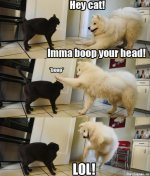Silver Member
Cat and dog

#### Attachments

•cat.jpg
89.7 KB · Views: 1,724

#### mosconiac

##### Job+Wife+Child=No Stroke
Silver Member#### mosconiac

##### Job+Wife+Child=No Stroke
Silver Member#### mosconiac

##### Job+Wife+Child=No Stroke
Silver Member#### mosconiac

##### Job+Wife+Child=No Stroke
Silver Member#### mosconiac

##### Job+Wife+Child=No Stroke
Silver MemberAnother not funny, but really cool one...#### mosconiac

##### Job+Wife+Child=No Stroke
Silver Member#### mosconiac

##### Job+Wife+Child=No Stroke
Silver Member#### mosconiac

##### Job+Wife+Child=No Stroke
Silver Member#### mosconiac

##### Job+Wife+Child=No Stroke
Silver Member#### mosconiac

##### Job+Wife+Child=No Stroke
Silver Member#### mosconiac

##### Job+Wife+Child=No Stroke
Silver MemberLast edited:

#### scooter_banks

Silver Member

##### "no buds chill"
Silver Member
wow there's like five different answers to that...what's funny is that I would think the teacher was trying to imply that B) is the right answer but it's not...lol

If you look at it from a perspective of a straight up random selection of 4 possible answers it's 25%.

However, 25% is two of the possible answers, so it would be 50%...

However, 50% is one of the possible answers also, so it would be 75%.

However, 75% is not a possible answer, so it would be 0%....

#### flash5153

##### none
Silver Member
what's funny is that I would think the teacher was trying to imply that B) is the right answer but it's not...lol

If you look at it from a perspective of a straight up random selection of 4 possible answers it's 25%.

However, 25% is two of the possible answers, so it would be 50%...

However, 50% is one of the possible answers also, so it would be 75%.

However, 75% is not a possible answer, so it would be 0%....

I see what you are saying but if a random draw on 4 possible answers ,,,the answer is 25%. It doesn't matter what the other choices are. That's a trap to read any other answer other than 25%. they mean nada.

#### purpdrag

##### AzB Silver Member
Silver Member
what's funny is that I would think the teacher was trying to imply that B) is the right answer but it's not...lol

If you look at it from a perspective of a straight up random selection of 4 possible answers it's 25%.

However, 25% is two of the possible answers, so it would be 50%...

However, 50% is one of the possible answers also, so it would be 75%.

However, 75% is not a possible answer, so it would be 0%....

The whole point of this one is that there is no correct answer given those choices. Made me think tho.

#### krupa

##### The Dream Operator
Silver Member
I see what you are saying but if a random draw on 4 possible answers ,,,the answer is 25%. It doesn't matter what the other choices are. That's a trap to read any other answer other than 25%. they mean nada.

The answers are not evenly distributed. There's a 50% chance of randomly choosing "25%" and 25% of choosing one of the other two.

#### stljohnny

##### knowledge > execution. :(
Silver Member
what's funny is that I would think the teacher was trying to imply that B) is the right answer but it's not...lol

If you look at it from a perspective of a straight up random selection of 4 possible answers it's 25%.

However, 25% is two of the possible answers, so it would be 50%...

However, 50% is one of the possible answers also, so it would be 75%.

However, 75% is not a possible answer, so it would be 0%....

I think your math might be a little off. You can't count the real answer into the question's hypothesis. You still have a 1 in 4 chance when answering randomly. But, because the values of two of the choices are the same, you could say you have a 2 in 4 chance to get in right. The answer is 50%.

#### KoolKat9Lives

##### Taught 'em all I know
Silver Member
I think your math might be a little off. You can't count the real answer into the question's hypothesis. You still have a 1 in 4 chance when answering randomly. But, because the values of two of the choices are the same, you could say you have a 2 in 4 chance to get in right. The answer is 50%.

The chance of you randomly choosing the 50% answer is 25%. The chance of you randomly picking one of the 25% answers is 50%.

Ain't no correct answer IMO! A real mind-bender! Like this guy: